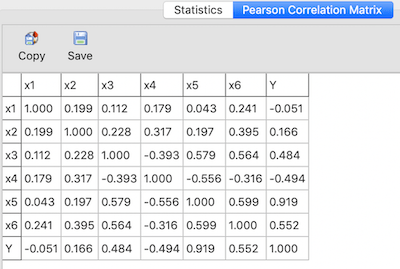# The detection of multicollinearity in ndCurveMaster

ndCurveMaster offers a multicollinearity detection option to improve the quality of models developed through the use of:

• a variance inflation factor (VIF),
• a Pearson Correlation Matrix.

to improve the quality of models which are developed.

### Variance Inflation Factor (VIF)

The VIF index is commonly used for the detection of multicollinearity (more details can be found here). There is no formal VIF value to determine the presence of multicollinearity. Any VIF values that exceed 10 are often regarded as an indication of multicollinearity, but in weaker model values above 2.5 this may be a cause for concern.
ndCurveMaster calculates the VIF values of each model. These VIF values are shown in the last column of the regression analysis table for each predictor, see below:In addition, ndCurveMaster offers a search facility for models with a VIF limit value. The user can select a “VIF cannot exceed” checkbox to only display models that do not exceed the selected VIF value. The default VIF limit value is 10, see below:The user can increase or lower the limit.

### Pearson Correlation Matrix

The Pearson Correlation Matrix window shows Pearson Correlation coefficients between each pair of variables:Examining the correlations of variables is the simplest way to detect multicollinearity. In general, an absolute correlation coefficient of more than 0.7 between two variables (or more) indicates the presence of multicollinearity.